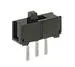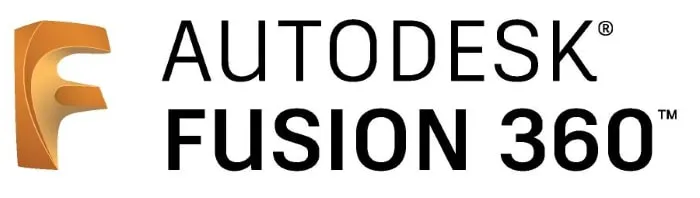Published © GPL3+

# Shaking Arduino Dice

One year ago I built a simple Arduino dice with my son. We have learned a lot in the meantime and it's time for an update...

BeginnerFull instructions provided4 hours9,956

## Things used in this project

### Hardware componentsArduino Nano R3
×1
 3mm 8x8 Dot Matrix MAX7219
×1
 Tilt switch module and a digital interface
×1Slide Switch
×1
 MT3608 DC 2A Step Up Power Module 2v-24v to 5v/9v/12v/28V Boost Converter
×1

### Software apps and online servicesArduino IDEAutodesk Fusion 360

### Hand tools and fabrication machinesSoldering iron (generic)Hot glue gun (generic)

Read more

## Code

### wuerfel8x8.ino

Arduino
Code for the shaking dice
```/*
Blog-Artikel: Schttel-Wrfel mit LED Matrix und Bewegungssimulation

https://techpluscode.de/schuettel-wuerfel-mit-led-matrix-und-bewegungssimulation/

techpluscode.de
Copyright 2019 von Thomas Angielsky
*/

//Bibliothek fr die Ansteuerung der 8x8 LED-Matrix einbinden
//Include lib for the 8x8 LED matrix
#include "LedControl.h"

int PinTiltX = 2; //Pin fr Tiltsensor X
int PinTiltY = 3; //Pin fr Tiltsensor Y

//Pins der LED-Matrix
//Pins of the LED matrix
int PinCLK = 7;
int PinCS = 6;
int PinDIN = 5;

LedControl lc = LedControl(PinDIN, PinCLK, PinCS, 1);

//Koordinaten der Wrfelaugen in der LED-Matrix
//Coordinates of the Dice points in the LED matrix
int DicePic =
{
{ //leere Matrix und Startposition:
{9,9}, //1. Punkt
{9,8}, //2. Punkt
{9,7}, //3. Punkt
{9,6}, //4. Punkt
{9,5}, //5. Punkt
{9,4} //6. Punkt
},
{ //1:
{4,4}, //1. Punkt
{-1,-1}, //2. Punkt
{-1,-1}, //3. Punkt
{-1,-1}, //4. Punkt
{-1,-1}, //5. Punkt
{-1,-1}  //6. Punkt
},
{ //2:
{2,2}, //1. Punkt
{6,6}, //2. Punkt
{-1,-1}, //3. Punkt
{-1,-1}, //4. Punkt
{-1,-1}, //5. Punkt
{-1,-1}  //6. Punkt
},
{ //3:
{2,6}, //1. Punkt
{6,2}, //2. Punkt
{4,4}, //3. Punkt
{-1,-1}, //4. Punkt
{-1,-1}, //5. Punkt
{-1,-1}  //6. Punkt
},
{ //4:
{2,2}, //1. Punkt
{2,6}, //2. Punkt
{6,2}, //3. Punkt
{6,6}, //4. Punkt
{-1,-1}, //5. Punkt
{-1,-1}  //6. Punkt
},
{ //5:
{2,2}, //1. Punkt
{2,6}, //2. Punkt
{6,2}, //3. Punkt
{6,6}, //4. Punkt
{4,4}, //5. Punkt
{-1,-1}  //6. Punkt
},
{ //6:
{2,1}, //1. Punkt
{2,4}, //2. Punkt
{2,7}, //3. Punkt
{6,1}, //4. Punkt
{6,4}, //5. Punkt
{6,7}  //6. Punkt
},
{ //Start:
{-1,-1}, //1. Punkt
{-1,-1}, //2. Punkt
{-1,-1}, //3. Punkt
{-1,-1}, //4. Punkt
{-1,-1}, //5. Punkt
{-1,-1}  //6. Punkt
}
};

//Variablen der Wrfelaugen: Position, Richtung, Geschwindigkeit fr X und Y
//Variables of the dice: position, direction, speed for X and Y
float DiceXpos;
float DiceXdir;
volatile byte DiceXspeed;
float DiceYpos;
float DiceYdir;
volatile byte DiceYspeed;

int DiceValue;
unsigned long timestamp;
byte Mode;
int volatile shakes;
int ShakesPerSecond;
int step;

void InterruptChecks() {
//Schttel-Anzahl zhlen
//Count Shakes
shakes=shakes+1;
//Serial.println(millis());
timestamp=millis();
}

void SetSpeedX() {
if (Mode==0) {
//alle Wrfel in X beschleunigen
//Speed-up dice in X
for (int i = 0; i < 6; i++) {
if (DiceXspeed[i]<255) {DiceXspeed[i]=DiceXspeed[i]+5;}
}
}
InterruptChecks();
}

void SetSpeedY() {
if (Mode==0) {
//alle Wrfel in Y beschleunigen
//Speed-up dice in Y
for (int i = 0; i < 6; i++) {
if (DiceYspeed[i]<255) {DiceYspeed[i]=DiceYspeed[i]+5;}
}
}
InterruptChecks();
}

void ShowLed(int x, int y, bool onoff) {
//LED nur anzeigen, wenn im sichtbaren Bereich
//show only, when x/y in matrix
if ((x<8) and (y<8) and (x>=0) and (y>=0)) {
lc.setLed(0, x, y, onoff);
}
}

void ShowDot(int x, int y, bool onoff) {
//Wrfel-Auge anzeigen oder ausblenden
//Show or hide dice point
ShowLed(x-1, y-1, onoff);
ShowLed(x, y-1, onoff);
ShowLed(x-1, y, onoff);
ShowLed(x, y, onoff);
}

void ShowDicePic(int value) {
//Wurf anzeigen
//Show dice

boolean done;

//alle Punkte von der aktuellen Position aus zur Zielposition von DiceValue bewegen
//move all points from current position to destination of DiceValue
for (int i = 0; i < 6; i++) {
DiceXspeed[i]=100;
DiceYspeed[i]=100;

//Werte fr X berechnen
//Calc x values
DiceXdir[i]=0;
if (int(DiceXpos[i])>DicePic[value][i]) {DiceXdir[i]=-1;}
else if (int(DiceXpos[i])<DicePic[value][i]) {DiceXdir[i]=1;}

DiceYdir[i]=0;
if (int(DiceYpos[i])>DicePic[value][i]) {DiceYdir[i]=-1;}
else if (int(DiceYpos[i])<DicePic[value][i]) {DiceYdir[i]=1;}
}

//Serial.println(value);
//Serial.println("Bewegung Start // Start moving");
//Punkte bewegen
do {
//Serial.println("Bewegung // Moving");
for (int i = 0; i < 6; i++) {
if (int(DiceXpos[i])!=DicePic[value][i]) {
DoStep(DiceXpos[i],DiceXdir[i],DiceXspeed[i],false);
}
if (int(DiceYpos[i])!=DicePic[value][i]) {
DoStep(DiceYpos[i],DiceYdir[i],DiceYspeed[i],false);
}
}

lc.clearDisplay(0);
for (int i = 0; i < 6; i++) {
ShowDot(int(DiceXpos[i]), int(DiceYpos[i]), true);
}

delay(50);

//Sind alle Augen an ihrer Zielposition
//Dice points are on destition position
done=true;
for (int i = 0; i < 6; i++) {
if (int(DiceXpos[i])!=DicePic[value][i]) {done=false;}
if (int(DiceYpos[i])!=DicePic[value][i]) {done=false;}
}

} while (done==false);
//Serial.println("Bewegung Ende // End moving");

lc.clearDisplay(0);
for (int i = 0; i < 6; i++) {
ShowDot(DicePic[value][i],DicePic[value][i], true);
}
}

void DoStep(float &pos, float &dir, volatile byte &sp, bool check) {
pos=pos+float(sp)/255*dir;

if (check==true) {
if (pos>7) {
pos=7;
dir=dir*(-1);
}
if (pos<1) {
pos=1;
dir=dir*(-1);
}

}
// Geschwindigkeit wird pro Schritt langsamer
// Velocity decreases every step
if (sp>0) {sp=sp-1;}
}

void MoveDots() {
//alle Wrfel einen Schritt weiter bewegen
//move dice points one step further
for (int i = 0; i < 6; i++) {
//neue Koordinaten berechnen
//calc new coordinates
DoStep(DiceXpos[i],DiceXdir[i],DiceXspeed[i],true);
DoStep(DiceYpos[i],DiceYdir[i],DiceYspeed[i],true);
}

//Wrfel-Augen anzeigen
//show dice points
lc.clearDisplay(0);
for (int i = 0; i < 6; i++) {
ShowDot(int(DiceXpos[i]), int(DiceYpos[i]), true);
}

}

void setup() {
//Der MAX7219 ist beim Starten im Power-Saving Modus,
//er muss aufgeweckt werden.
//The MAX7219 is in power-saving mode on startup,
//we have to do a wakeup call
lc.shutdown(0, false);
//Helligkeit auf einen Mittelwert
//Set the brightness to a medium values
lc.setIntensity(0, 8);
//und Display lschen
//and clear the display
lc.clearDisplay(0);

randomSeed(analogRead(0));
DiceValue=0;

for (int i = 0; i < 6; i++) {
DiceXpos[i]=DicePic[i];
DiceYpos[i]=DicePic[i];

DiceXdir[i]=random(3)-1;
DiceYdir[i]=random(3)-1;
DiceXspeed[i]=random(126)+120;
DiceYspeed[i]=random(126)+120;
}

//Pins einstellen
//Setup the pins
pinMode(PinTiltX, INPUT_PULLUP);
pinMode(PinTiltY, INPUT_PULLUP);
attachInterrupt(digitalPinToInterrupt(PinTiltX),SetSpeedX,CHANGE);
attachInterrupt(digitalPinToInterrupt(PinTiltY),SetSpeedY,CHANGE);

lc.clearDisplay(0);

timestamp=millis();
Mode=1;

ShowDicePic(6);
delay(1000);

lc.clearDisplay(0);
Mode=0;
Serial.begin(9600);
step=0;
shakes=0;
}

void loop() {
delay(50);
step=step+1;
if (step>20) {
//1 sek ist vorbei
//1 sec is over
step=0;
ShakesPerSecond=shakes;
shakes=0;
}

if (Mode==0) {
MoveDots();
if (millis()-timestamp>2000) {
//seit 2 sek kein Schtteln mehr
//there is no shaking since 2 sec
Mode=1;
DiceValue=random(6)+1;
ShowDicePic(DiceValue);
}
}

if (ShakesPerSecond>5) {
//Es wird wieder geschttelt
//shaking again
Mode=0;
}

}
```

## Credits

### Thomas Angielsky

15 projects • 17 followers
Mechanical engineer, maker, love woodwork, like Lazarus